Geometry

#### Challenge Quizzes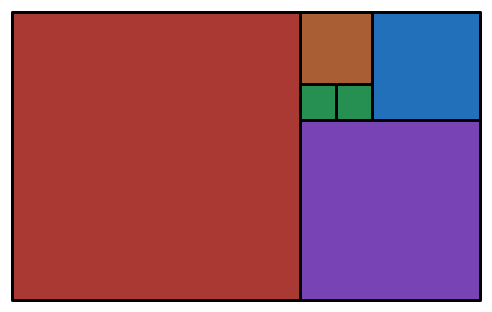In the above rectangle, all the smaller shapes are squares. If the two green squares in the center each have a side length of 1, what is the area of the rectangle?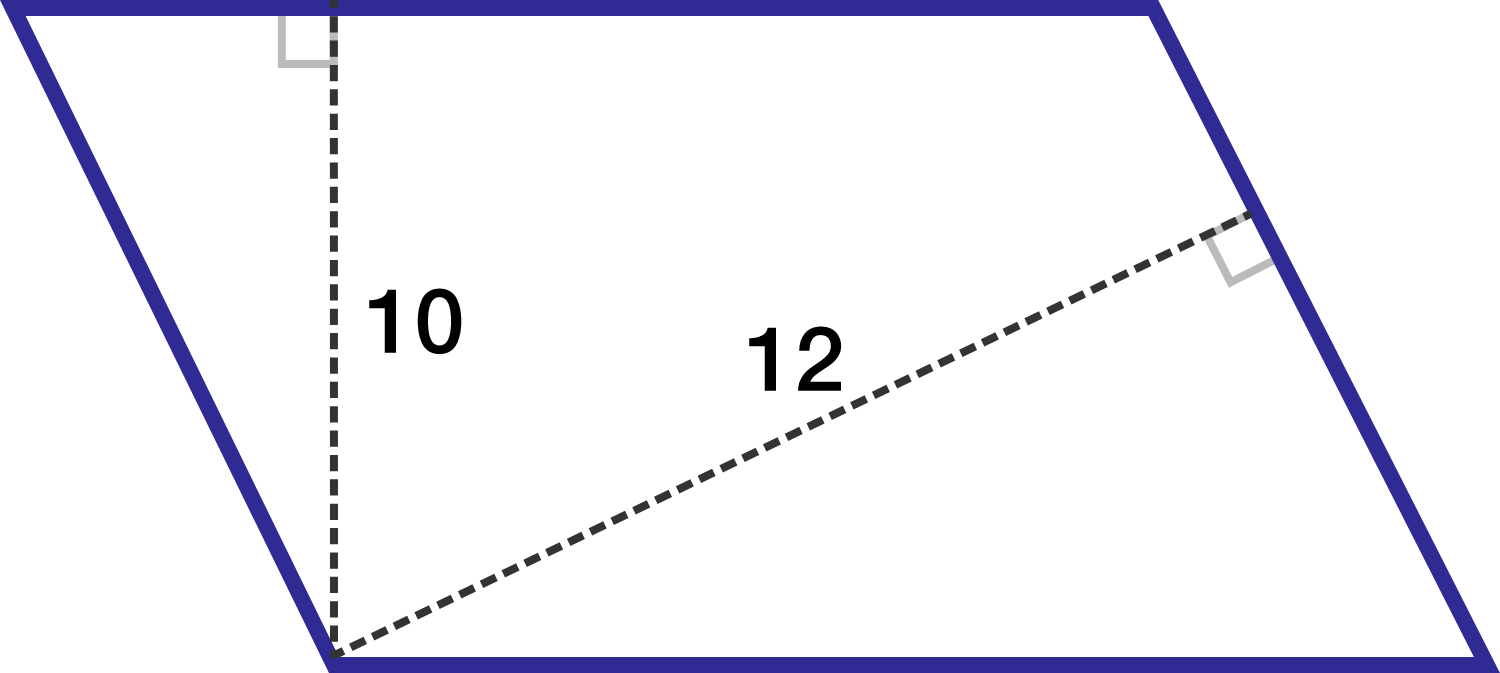The lengths of the altitudes from a vertex of the parallelogram to the other two sides are 10 and 12.

If the parallelogram has a perimeter of 176, find the area.

Note: The figure is not drawn to scale.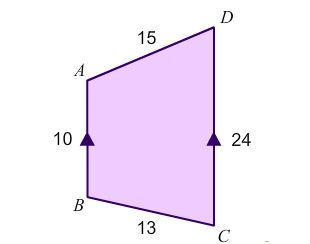$ABCD$ is a quadrilateral with measurements of its sides shown in the above diagram. If $AB$ is parallel to $CD$. What is the area of the quadrilateral?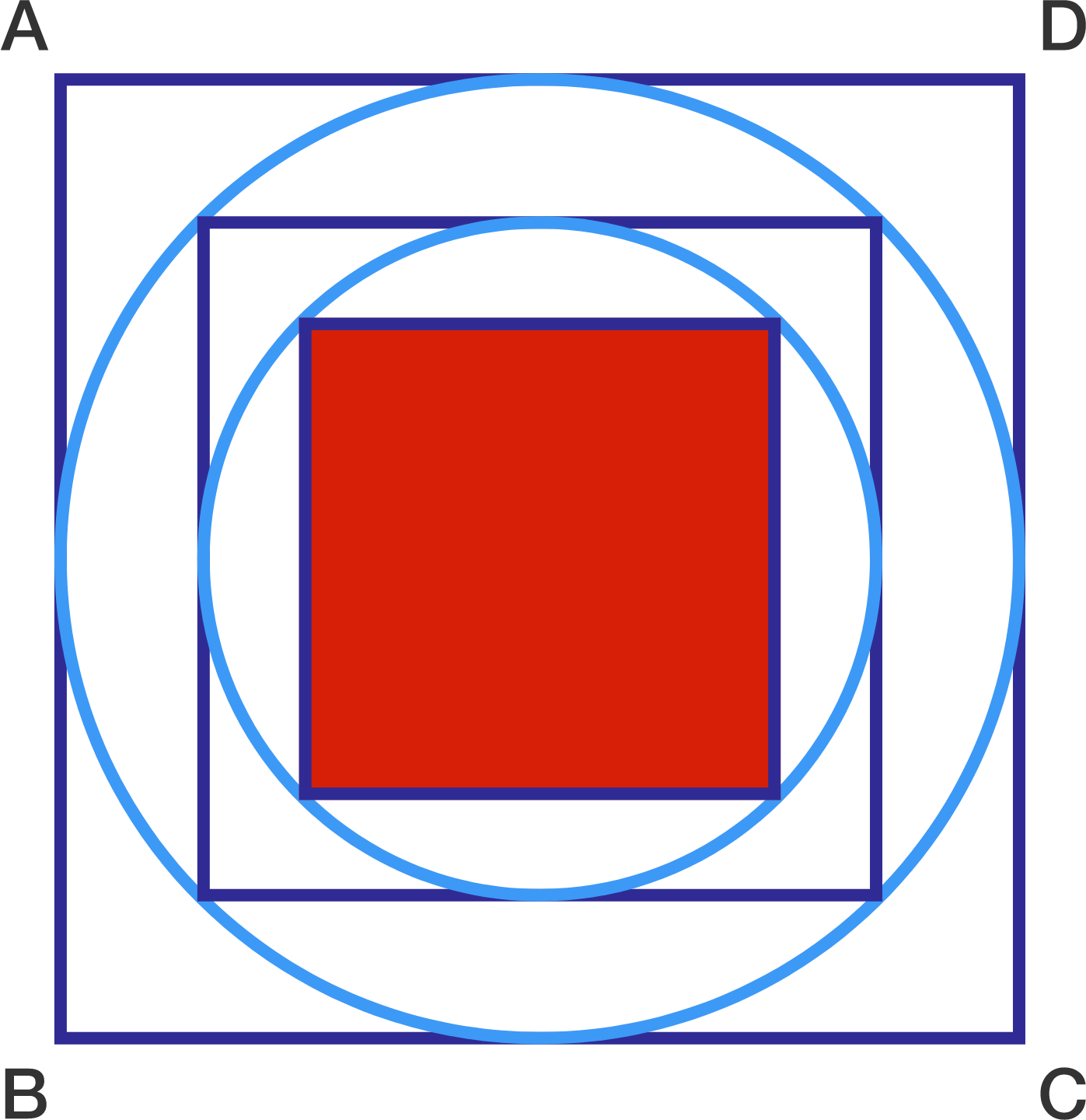If all of the rectangles in the figure are squares and the area of the shaded square is 25, find the area of square ABCD.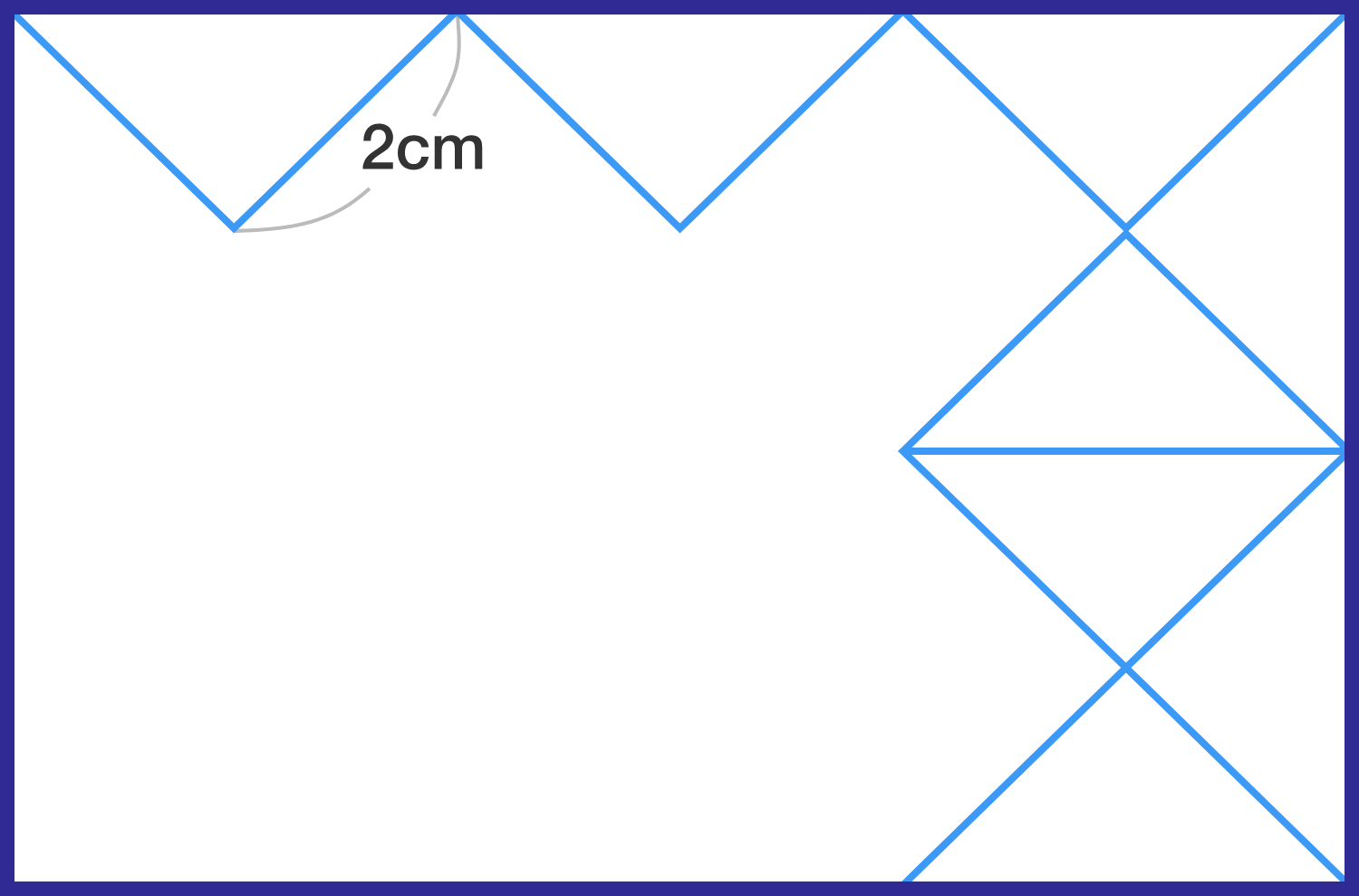If the triangles are isosceles, what is the area of the quadrilateral?

×